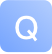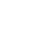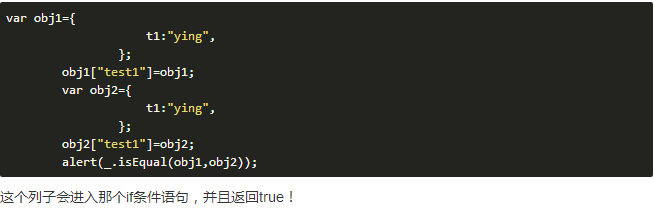# underscore.js 内部方法 eq(a,b,aStack,bStack)-问答-阿里云开发者社区-阿里云## underscore.js 内部方法 eq(a,b,aStack,bStack)

`````` // Internal recursive comparison function for `isEqual`.
var eq = function(a, b, aStack, bStack) {
// Identical objects are equal. `0 === -0`, but they aren't identical.
// See the [Harmony `egal` proposal](http://wiki.ecmascript.org/doku.php?id=harmony:egal).
if (a === b) return a !== 0 || 1 / a === 1 / b;
// A strict comparison is necessary because `null == undefined`.
if (a == null || b == null) return a === b;
// Unwrap any wrapped objects.
if (a instanceof _) a = a._wrapped;
if (b instanceof _) b = b._wrapped;
// Compare `[[Class]]` names.
var className = toString.call(a);
if (className !== toString.call(b)) return false;
switch (className) {
// Strings, numbers, regular expressions, dates, and booleans are compared by value.
case '[object RegExp]':
// RegExps are coerced to strings for comparison (Note: '' + /a/i === '/a/i')
case '[object String]':
// Primitives and their corresponding object wrappers are equivalent; thus, `"5"` is
// equivalent to `new String("5")`.
return '' + a === '' + b;
case '[object Number]':
// `NaN`s are equivalent, but non-reflexive.
// Object(NaN) is equivalent to NaN
if (+a !== +a) return +b !== +b;
// An `egal` comparison is performed for other numeric values.
return +a === 0 ? 1 / +a === 1 / b : +a === +b;
case '[object Date]':
case '[object Boolean]':
// Coerce dates and booleans to numeric primitive values. Dates are compared by their
// millisecond representations. Note that invalid dates with millisecond representations
// of `NaN` are not equivalent.
return +a === +b;
}

var areArrays = className === '[object Array]';
if (!areArrays) {
if (typeof a != 'object' || typeof b != 'object') return false;

// Objects with different constructors are not equivalent, but `Object`s or `Array`s
// from different frames are.
var aCtor = a.constructor, bCtor = b.constructor;
if (aCtor !== bCtor && !(_.isFunction(aCtor) && aCtor instanceof aCtor &&
_.isFunction(bCtor) && bCtor instanceof bCtor)
&& ('constructor' in a && 'constructor' in b)) {
return false;
}
}
// Assume equality for cyclic structures. The algorithm for detecting cyclic
// structures is adapted from ES 5.1 section 15.12.3, abstract operation `JO`.
var length = aStack.length;
while (length--) {
// Linear search. Performance is inversely proportional to the number of
// unique nested structures.
if (aStack[length] === a) return bStack[length] === b;
}

// Add the first object to the stack of traversed objects.
aStack.push(a);
bStack.push(b);
var size, result;
// Recursively compare objects and arrays.
if (areArrays) {
// Compare array lengths to determine if a deep comparison is necessary.
size = a.length;
result = size === b.length;
if (result) {
// Deep compare the contents, ignoring non-numeric properties.
while (size--) {
if (!(result = eq(a[size], b[size], aStack, bStack))) break;
}
}
} else {
// Deep compare objects.
var keys = _.keys(a), key;
size = keys.length;
// Ensure that both objects contain the same number of properties before comparing deep equality.
result = _.keys(b).length === size;
if (result) {
while (size--) {
// Deep compare each member
key = keys[size];
if (!(result = _.has(b, key) && eq(a[key], b[key], aStack, bStack))) break;
}
}
}
// Remove the first object from the stack of traversed objects.
aStack.pop();
bStack.pop();
return result;
};

// Perform a deep comparison to check if two objects are equal.
_.isEqual = function(a, b) {
return eq(a, b, [], []);
};
// Assume equality for cyclic structures. The algorithm for detecting cyclic
// structures is adapted from ES 5.1 section 15.12.3, abstract operation JO.
var length = aStack.length;
while (length--) {
// Linear search. Performance is inversely proportional to the number of
// unique nested structures.
if (aStack[length] === a) return bStack[length] === b;
}
``````

1 条回答写回答取消 提交回答
• 小旋风柴进2019-07-17 19:05:12
赞同 展开评论 打赏

285
1
0
305
1
0
3134
1
0
1422
1
0
1708
1
0
1602
4
0
1163
1
0
1966
2
0
1518
1
0
1999
1
0

JavaScript函数